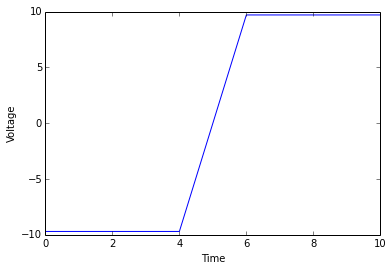# Chapter 5 : Comparators and Waveform generators¶

## Example 5.1 Page No.212¶

In :
%matplotlib inline
import matplotlib.pylab as plt
import numpy as np
# Given Data

Vz1 = 9.0
Vz2 = 9.0

x  = np.array([-1, -1, -1, -1, -1, 0, 1, 1, 1, 1, 1])
plt.plot(9.7*x)
plt.xlabel('Time')
plt.ylabel('Voltage')
plt.show()## Example 5.2 Page No.215¶

In :
# Given data

R2 = 100.0
R1 = 50.0*10**3
Vref = 0
vi = 1
Vsat = 14.0

# Solution

VUT = (R2*Vsat)/(R1+R2)
VLT = (R2*-Vsat)/(R1+R2)

# Displaying the values

print "The value of Upper threshold voltage =  ",int(round(VUT*10**3,0)),"mV"
print "The value of Lower threshold voltage = ",int(round(VLT*10**3,0)),"mV"

The value of Upper threshold voltage =   28 mV
The value of Lower threshold voltage =  -28 mV


## Example 5.3 Page No.216¶

In :
import numpy as np
# Given data

VUT = 0
VH = 0.2
f = 10.0**3
# Solution

VLT = VUT - VH
theta = round(np.arcsin(VH/2),1)
T = 1/f
Ttheta = theta/(2*np.pi*f)
T1 = (T/2) + Ttheta
T2 = (T/2) - Ttheta

# Displayig the values

print "The value of Lower threshold voltage = ",VLT,"V"
print "The value of angle theta             = ",theta,"Radian"
print "The value of Timeperiode             = ",round(Ttheta*10**3,3),"ms"
print "The value of T1                      = ",round(T1*10**3,3),"ms"
print "The value of T2                      = ",round(T2*10**3,3),"ms"

The value of Lower threshold voltage =  -0.2 V
The value of angle theta             =  0.1 Radian
The value of Timeperiode             =  0.016 ms
The value of T1                      =  0.516 ms
The value of T2                      =  0.484 ms


## Example 5.4 Page No.225¶

In :
import math
# Given data

f = 100
C = 0.1*10**-6

# Solution

R = 1/(math.sqrt(6)*2*math.pi*(10**-7)*100)
R1 = 10*R
Rf = 29*R1

# Displaying the solutions

print "The value of R  =",round(R*10**-3,3),"Kilo Ohms"
print "The value of R1 =",int(round(R1*10**-3,0)),"Kilo Ohms"
print "The value of Rf =",int(round(Rf*10**-3,0)),"Kilo Ohms"

The value of R  = 6.497 Kilo Ohms
The value of R1 = 65 Kilo Ohms
The value of Rf = 1884 Kilo Ohms

In [ ]: